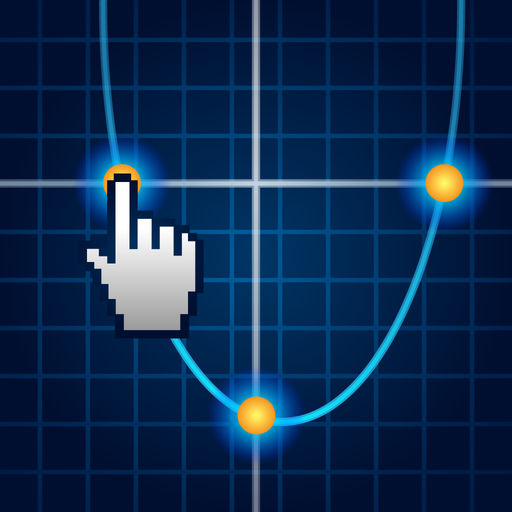MathDraw: Draw, Chart, Plot, Graph, Calc Math Equations. Linear, Quadratic and Trigonometry. Bhaskara Formula. Perfect for College and University.
Removed from the App Store# MathDraw: Draw, Chart, Plot, Graph, Calc Math Equations. Linear, Quadratic and Trigonometry. Bhaskara Formula. Perfect for College and University.

## Carlos Lima Jr

3.3.5 for iPhone, iPad
Free
0

0 Ratings

Update Date

2015-07-10

Size

44.1 MB

### Screenshots for iPhone

iPhone
More Screenshots for iPad
MathDraw: Draw, Chart, Plot, Graph, Calc Math Equations. Linear, Quadratic and Trigonometry. Bhaskara Formula. Perfect for College and University. Description
This simple and easy app is essential for any math student. Draw, chart, graph and plot linear, quadratic, trigonometry and custom equations. Get quadratic roots. Learn and understand math through graphs and equations. This app It is unique because it allows the user to interact with the graphic layout, enabling drag vertices and intersection points, and get in real-time the changes that occur in the equation coefficients.

## Key Features ##

- Several examples of mathematical equations with charts of the most dif, ferent ways;
- Module for the assembly of equations f (x). Allows the use of parentheses, basic mathematical operators (+, -, *, /) and many mathematical functions such as sin, cos, tan, ln, sqrt, cube, abs, acos, asin, atan;
- Module for the construction and analysis of linear equations, enabling the drag of all the points of intersection between the line and the graphics axes;
- Module for the construction and analysis of quadratic equations, enabling drag the vertex of the parabola, and the parable of the intersection points with the graphics axes;
- Module for trigonometric analysis.
More Information
Price:
Free
Version:
3.3.5
Size:
44.1 MB
Update Date:
2015-07-10
Developer:
Carlos Lima Jr
Language:
Chinese Czech Dutch English French German Italian Japanese Korean Polish Portuguese Russian Spanish Swedish Turkish
More
You May Also Like
Developer Apps